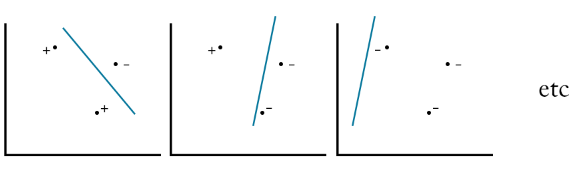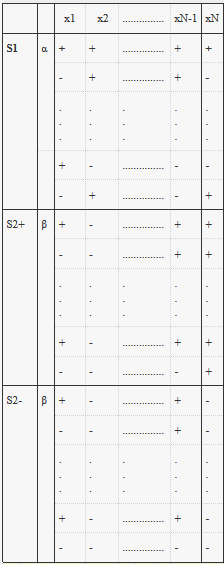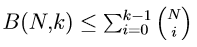2022-01-01
###### Understanding AdaBoost algorithm and its mathematics
2022-01-23

The size of our data set N plays a major role when it comes to the reliability of the generalization Ein ~ Eout. Also, we can’t use all of our data for training purposes only, simply because there won’t be any data left to estimate the out-of-sample error. Consequently, we have to be mindful of not only how we use data but also of the complexity of the used model, since more parameters mean bigger hypotheses set and therefore a looser generalization bound (A bigger value of M in the bound in Hoeffding’s inequality). In this article, we will revise our previous definition of generalization and see how it applies in a training context and then in testing.

# Out of sample error

We know that even the rarest events will occur if we try an experiment indefinitely (even a broken clock is right twice a day!). We, therefore, had to account for this problem in Hoeffding’s inequality because, during training, the learning algorithm tries a large number of different hypothesis functions before finding the best one. We consequently added a penalization to the inequality in order to account for this:

card(H) = M.

However in testing, we only have the one best hypothesis that minimizes Ein and we want to check how well it performs on the data set, therefore M=1 and Hoeffding’s inequality becomes more of a “verification” step than a learning process:

# Infinite hypotheses spaces and Hoeffding’s inequality

In practical situations though, the hypotheses space often has an infinite dimension (ex: the space of possible regression lines, there is an infinite number of lines in a plane to choose from). Consequently, goes to ∞ and Hoeffding’s inequality in training becomes:

And clearly, a probability being bound by infinity is no good for generalization because we will have no clue how far Ein(h) is deviating from Eout(h). But does this mean that learning is not feasible in an infinite hypothesis space? No, and the ingenious solution to this problem is dichotomies!

A dichotomy is a “sub-space” of the original hypotheses space that contains a set of “similar” hypotheses (similar hypotheses are grouped into dichotomies). A hypothesis h₁ is similar to h₂ if when applied to a data set D, they will result in the same output or classification for every data point:

h₁, h₂, and every other hypothesis that separates the data the same way form a dichotomy and the brilliant idea is that instead of trying every possible hypothesis (which is impossible since H is infinite), we only go through the possible dichotomies, which will allow us to avoid using (the number of possible hypotheses = ∞) in the generalization bound and instead use a finite quantity related to the number of possible dichotomies.

# The growth function

Let D be our data set of size N.

What a growth function does is count the maximum number of dichotomies possible on N points: given N data points, the growth function places them in the X space in a way that’ll result in as many dichotomies as possible. The reason why the growth function does not count the possible dichotomies in our data set is that since we don’t know what’s the underlying probability distribution of D, we need to be “prepared” for the worst-case scenario, i.e, the data is coming from a probability distribution that has the highest number of dichotomies).

The formula counts the maximum number of possible dichotomies in by applying every possible hypothesis h in to the data, and whenever a new combination of points is discovered (different from the old discovered ones), the growth function increases by 1.

When all dichotomies are possible on N points, we say that we shattered the points using the hypotheses space.

The question now is are we allowed to replace the quantity with the growth function? If so, will that help our model’s generalization?

# Break Points

A break point is the minimal number of data points that a hypothesis set will fail to shatter. For example, in the next figure we can see that a perceptron can shatter at most 3 points, this means that no perceptron can give you all combinations in a 4 points data set, i.e, 4 is break point for the perceptrons hypotheses set:

However in the case of three points, for every possible combination, we can find a dichotomy that will have that combination as output:There’s a line that’ll separate the three points in any combination we want

We conclude that if no break points exist, then all data combinations are possible (all dichotomies are possible), and since we’re in the context of binary classification (either + or -, 1 or -1, etc) we will have:

On the other hand, if a break point k does exist then the growth function is going to be a polynomial function that will be dominated by the exponential in Hoeffding’s inequality.

We’ve established that there is still hope of generalization even in hypotheses’ spaces that are infinite in dimension. For this purpose, we’ve introduced the notions of dichotomies and growth functions that both make the generalization bound a lot friendlier (the upper bound in Hoeffding’s inequality). Before we continue I’d like to remind you that if is a break point, then for any points in our data, it is impossible to get all possible combinations (2^k).

Our goals now are, first, to prove that if a break point exists, the growth function is going to be upper-bounded by a polynomial:

If this is the case, then as N gets bigger we will get:

Due to the fact that exponentials dominate polynomials.

Second, we need to verify if we’re allowed to replace the number of possible hypotheses M in the generalization bound with the growth function.

# Part I

## Simple case

Without specifying any hypothesis set, for illustration purposes, let’s first consider the following example where we have three data points N = 3 and a break point k =2, this means that for any two points, we can’t have all 4 possible combinations: (+,+), (-,-), (+,-) (-,+). As a consequence, the number of combinations we can get on our three points is limited as well:

These combinations are allowed since no two points have all possible combinations.

If we add the last row, the highlighted cells give us all 4 combinations of the points x2 & x3, which is not allowed by the break point.

By the same logic, we can verify that the maximum number of possible combinations in the case of N=3 & k =2 is 4 (any new combination added to the first table will violate the condition of k = 2).

Also, we notice that if we remove x3, the highlighted cells result in two duplicate rows, and by simply adding x3, one time being “-” and the other “+”, we get 2 rows that are different.

This means that in any similar matrix, we have a group of unique rows that get their “uniqueness” via a certain xN (x3 in the example). We will call that group of rows S2 in what follows.

On the other hand, there’s a group of rows that are unique independently of xN, and they either occur with xN being “-” or “+” and not both. This group corresponds to S1 in the following section.

## Bounding the growth function

Let’s define the quantity B(N,k) that counts the maximum number of possible combinations on N points with k being a breakpoint (B(3,2) = 4 in the previous example).

B(N,k) corresponds to the number of rows in the following table:Table of possible combinations for N points with a k break point

Let α be the count of rows in the S1 group. We also divide the group S2 into S2+ where xN is a “+” and S2- where xN is “-” and each of them has β rows. This means that:

B(N,k) α + 2β : (0).

The purpose of the following steps is to find the recursive bound of B(N,k) (a bound defined by on different values of N & k).

For this purpose, we’ll start by trying to estimate α β which is the number of rows in the table without point xN and the group S2-. The result is a sub-table where all rows are different since the rows in S1 are inherently different without xN, and the rows in S2+ are different from the ones in S1 because if that wasn’t the case, the duplicate version of that row in S1 would get its “uniqueness” from xN and forcing it to leave S1 and join S2 (just like we’ve seen in the simple case example).

Furthermore, since in the bigger table (N points) there are no k points that have all possible combinations, it is impossible to find all possible combinations in the smaller table (N-1 points). This implies that k is a break point for the smaller table too.

Which will give us: α β < B(N-1,k) : (2).

The next step now is to find an estimation of β by studying the group S2 only and without the xN point:

Because the rows in S2+ are different from the ones in S2- only thanks to xN, when we remove xN, S2+ becomes the same as S2-.

Consequently, we will only focus on S2+.

For this smaller version of the original table, if we suppose that k is its break point, then we can find k-1 points that exist in all possible combinations. And if this is the case, when we add xN back, in both forms “-” and “+”, we get a table where we have all possible combinations of k points which is impossible since k is the breaking point. Therefore, we conclude that k-1 is in fact a break point for S2+. And since B(N-1, k-1) is the maximum number of combinations for N-1 that have a break point of k-1, we conclude that β < B(N-1, k-1) : (2).

(1) + (2) results in:

B(N,k) α 2β ≤ B(N-1, k) + B(N-1, k-1) (*)

One last thing we need to verify is that thanks to the recursive relationship (*) we can fill the following table of the values of for different values of and k:

Examples:

• k=1 and N=1, no one point can have all possible combinations, this means this point is either “+” or “-”.
• k=1 and N=5, every point can either be “+” or “-”, this means that only one combination is possible because as soon as we add a second combination that’s different from the first, we change the value of at least one of the points, which is contradictory to k=1.
• k=2 and N=3, this is the case in our first example (Simple case), and we’ve seen that indeed B(3,2) = 4.

## Theorem:The sum of N choose i, with i ranging from 0 to k, is a bound to B(N,k)

## Proof:

1. Boundary conditions: We can easily verify that for N=1 and k = 1, we have B(1,1) = 1 ≤ than 1 choose 0 = 1.
2. Induction: Let’s suppose we have

And remind the combinatorial Lemme where:

Finally, if we apply the recursive bound B(N,k) ≤ B(N-1, k) + B(N-1, k-1) to B(N+1, k) we get:

1. In the third line, we changed the range of from [0 -> k-2] to [1->k-1]and use i-1 instead
2. In the fourth line, we extracted the N choose 0 (=1) from the sum.
3. Finally, we use the combinatorial Lemme and add 1 back to the sum.
4. We will call the last sum

This is good news because since k is fixed, the combinatorial quantity that bounds B(N,k) is gonna be a polynomial in because if we develop that sum the maximum power is going to be N to the power of k-1, for i = k-1

## Bound of the growth function

We close this first part with the fact that if, for any hypotheses’ space H, a break point k exists, we have:

This is true because B(N,k) is the maximum number of possible combinations of N points independently of how many they are and also of the hypotheses’ space we’re studying (the growth function depends on the space). Consequently, B(N,k) is bigger than or equal to the growth function for any value of N.

Therefore:

# Part II

In this section, I will illustrate the main ideas needed to understand the proof of the theorem that allows us to use the growth function in Hoeffding’s inequality. Also, for a better understanding of this, I really advise you to watch the lecture at least starting from the 45th to the 60th minute. You can find the full proof here.

## The overlaps of false positive

! When our best hypothesis is in reality a bad approximation of the target function (because of the multiple testing), we will call this event a “false positive”.

We’ve seen before that the union bound was added to Hoeffding’s inequality to take into account the multiple testing problem that occurs when we go through a hypotheses’ set searching for the best hypothesis.

However, in the previous inequality, the generalization bound often goes to infinity, not only because most of the hypotheses’ sets are infinite (M->∞), but also because the union bound assumes that the probabilities in Hoeffding’s inequality related to the different hypotheses do not overlap. But, in reality, all hypotheses that classify the data, in the same way, will result in probabilities of Ein deviating from Eout that are very related to one another. Therefore, it makes sense to not try every possible hypothesis and instead try the possible dichotomies instead (all hypotheses in a dichotomy will classify the data the same way). We therefore get:

The growth function takes care of the redundancy of hypotheses that result in the same classification. Hence, if we are trying dichotomies instead of hypotheses, and are unlucky to get a false positive, this false positive include all the false positives we could’ve fallen into if we tried every hypothesis that belongs to this dichotomy. This not only reduces the generalization bound tremendously but most importantly makes it a finite quantity.

## But dichotomies do not relate to Eout?

The last part of the proof relates to the fact that the probability of a false positive occurring also depends on Eout, and Eout does not relate to the dichotomy that contains the best hypothesis, but on the hypothesis itself. This is not convenient since we’ve built our argument on dichotomies and not hypotheses.

To get around this problem, instead of computing just one in sample error Ein, we apply our hypothesis on two different data sets of the same size, and get Ein and E’in. And the idea is since both Ein and E’in are approximations of Eout, Ein will approximate E’in. (ex: if we try to approximate the average salary of data scientists in France by computing the average salary on two different data sets of the same size, we expect both computed values to be close to the population’s average salary but also be close to each other).

Now since our problem was losing the accuracy of Hoeffding’s inequality because of multiple testing, that same problem is going to occur nearly in the “same amount” when we try to track E’in instead of Eout. Finally, since we are not going to use Eout, we will be able to find a bound that has the growth function in it and that is legit to use.

The new resulting inequality is called the Vapnik-Chervonenkis (VC) Inequality and is as follows: